CAT  >  SNAP Mock Test - 4 (New Pattern)

# SNAP Mock Test - 4 (New Pattern) - CAT

Test Description

## 60 Questions MCQ Test Mock Test Series for SNAP - SNAP Mock Test - 4 (New Pattern)

SNAP Mock Test - 4 (New Pattern) for CAT 2023 is part of Mock Test Series for SNAP preparation. The SNAP Mock Test - 4 (New Pattern) questions and answers have been prepared according to the CAT exam syllabus.The SNAP Mock Test - 4 (New Pattern) MCQs are made for CAT 2023 Exam. Find important definitions, questions, notes, meanings, examples, exercises, MCQs and online tests for SNAP Mock Test - 4 (New Pattern) below.
Solutions of SNAP Mock Test - 4 (New Pattern) questions in English are available as part of our Mock Test Series for SNAP for CAT & SNAP Mock Test - 4 (New Pattern) solutions in Hindi for Mock Test Series for SNAP course. Download more important topics, notes, lectures and mock test series for CAT Exam by signing up for free. Attempt SNAP Mock Test - 4 (New Pattern) | 60 questions in 60 minutes | Mock test for CAT preparation | Free important questions MCQ to study Mock Test Series for SNAP for CAT Exam | Download free PDF with solutions
 1 Crore+ students have signed up on EduRev. Have you?
SNAP Mock Test - 4 (New Pattern) - Question 1

### Directions: Rearrange the following five sentences (A), (B), (C), (D) and (E) in the proper sequence to form a meaningful paragraph and then answer the question.A. But many of the currently-used triggers, like antibiotics, can have negative side effects or are unsafe for prolonged use.B. In recent years, scientists have worked to refine how cell therapies — which have shown promise in treating diseases like cancer — are controlled.C. In search of a better trigger, lead author Jianli Yin and her colleagues looked for a compound that was natural, nontoxic and perhaps even beneficial to health.D. These therapies often rely on a chemical compound to trigger cells to secrete a specific therapeutic substance.E. In addition to its laundry list of purported health benefits, green tea contains a molecule called protocatechuic acid, or PCA.Which of the following is the FIRST sentence of the sequence?

Detailed Solution for SNAP Mock Test - 4 (New Pattern) - Question 1
Point B introduces the subject - "cell therapies". All the other sentences relate to it. So, it should be the introductory sentence.

Point D mentions "these therapies" which relate to "cell therapies" introduced in point B.

Point D mentions "... chemical compound to trigger cells ...", which has been further mentioned in point A as "But many of the currently-used triggers ...".

So, A should come after D as it presents a contrast to D.

Point C mentions "In search of a better trigger", which relates to "negative side effects or are unsafe" in A. So, C should be the next sentence.

Point E should be placed after C as it mentions "list of purported health benefits", which relates to "beneficial to health" in C.

So, the correct order should be BDACE.

SNAP Mock Test - 4 (New Pattern) - Question 2

### Directions: Select the appropriate conjunction from the given choices to fill in the blank._______ you speak the truth, you will be dismissed.

Detailed Solution for SNAP Mock Test - 4 (New Pattern) - Question 2
In this conditional statement, 'unless', which means 'if not', should be used. 'If' does not fit well with the meaning of the sentences. Being 'dismissed' on speaking the 'truth' goes against the general conventions and the implied meaning of the sentence.
SNAP Mock Test - 4 (New Pattern) - Question 3

### Directions: Select the appropriate conjunction from the given choices to fill in the blank._______ you have not obeyed me, I will not make anymore suggestions.

Detailed Solution for SNAP Mock Test - 4 (New Pattern) - Question 3
As the reason is already known, the correct usage will be 'since'. Double negatives are not used, so options 3 and 4 are incorrect.
SNAP Mock Test - 4 (New Pattern) - Question 4

Directions: Select the word which is the synonym of the given word/ underlined word.

Precocious

Detailed Solution for SNAP Mock Test - 4 (New Pattern) - Question 4
'Precocious' means exceptionally smart, ahead of age in understanding. So, option 2 is the correct answer.
SNAP Mock Test - 4 (New Pattern) - Question 5

Directions: Choose the correctly spelt word.

Detailed Solution for SNAP Mock Test - 4 (New Pattern) - Question 5
Correctly spelt word is 'pungent,' which refers to a smell that is strong and sharp.
SNAP Mock Test - 4 (New Pattern) - Question 6

Directions: Choose the alternative that best describes the meaning of the underlined idiom/phrase.

I find her very annoying as she has the awful habit of poking her nose into my affairs.

Detailed Solution for SNAP Mock Test - 4 (New Pattern) - Question 6
'To poke one's nose into' means to interfere in something.
SNAP Mock Test - 4 (New Pattern) - Question 7

Directions: Choose the appropriate word from the given options and fill in the blank.

The incidence has brought the ___________ within the ruling party into sharper focus.

Detailed Solution for SNAP Mock Test - 4 (New Pattern) - Question 7
''Squabbles'' is the correct answer, which means ''petty arguments''. All the other options make the sentence illogical.
SNAP Mock Test - 4 (New Pattern) - Question 8

Directions: Fill in the blank with a suitable idiom/phrase.

The monetary help arrived too late. He was already on his ______________.

Detailed Solution for SNAP Mock Test - 4 (New Pattern) - Question 8
If one is on his last legs, one is in a very weak condition or about to die. Option 1 is correct.

The monetary help arrived too late. He was already on his last legs.

SNAP Mock Test - 4 (New Pattern) - Question 9

Choose the option that best replaces the phrase in underline without changing the meaning of the sentence below:

The kids had a whale of a time at the swimming pool.

Detailed Solution for SNAP Mock Test - 4 (New Pattern) - Question 9
"A whale of a time" refers to an exciting experience.

Hence, the answer is option C.

SNAP Mock Test - 4 (New Pattern) - Question 10

Select the option that is closest in meaning to the word given below:

Sardonic

Detailed Solution for SNAP Mock Test - 4 (New Pattern) - Question 10
’Sardonic’ means Cynical or grim. Therefore, option B is the right answer.
SNAP Mock Test - 4 (New Pattern) - Question 11

Identify the figure of speech.

Fear gripped the students waiting for their exam results.

Detailed Solution for SNAP Mock Test - 4 (New Pattern) - Question 11
In the given sentence, a non-human entity "Fear" is given the human ability of "gripping".

Thus, the figure of speech used is Personification.

SNAP Mock Test - 4 (New Pattern) - Question 12

Identify the figure of speech.

Fear gripped the students waiting for their exam results.

Detailed Solution for SNAP Mock Test - 4 (New Pattern) - Question 12
In the given sentence, a non-human entity "Fear" is given the human ability of "gripping".

Thus, the figure of speech used is Personification.

SNAP Mock Test - 4 (New Pattern) - Question 13

A sentence is given in direct speech. Choose the option that represents the same sentence in indirect speech:

Balu said to his students ‘Try to achieve what you dream’.

Detailed Solution for SNAP Mock Test - 4 (New Pattern) - Question 13
While converting from direct to indirect speech, ‘said to’ must be converted to ‘told’. The inverted commas must be removed. Also, the statement within the inverted commas must be devoid of first or second person pronouns.

The correct form is Balu told his students to try to achieve what they dream of.

Therefore, option B is the right answer.

SNAP Mock Test - 4 (New Pattern) - Question 14

Choose the option which gives the meaning of the below-given mania/phobia.

Nomophobia

Detailed Solution for SNAP Mock Test - 4 (New Pattern) - Question 14
Nomophobia is an abbreviation of "No Mobile Phone Phobia" and refers to the fear of being without one's mobile phone.

Hence, the answer is option A.

SNAP Mock Test - 4 (New Pattern) - Question 15

Identify the source of the phrase given below.

"De Facto"

Detailed Solution for SNAP Mock Test - 4 (New Pattern) - Question 15
The phrase "De Facto" means "in fact".

It has its origins in the Latin language.

SNAP Mock Test - 4 (New Pattern) - Question 16

What will come in place of the question mark (?) in the following number series?

10, 65, 626, 7777, ?

Detailed Solution for SNAP Mock Test - 4 (New Pattern) - Question 16
The pattern is

32 + 1 = 10

43 + 1 = 65

54 + 1 = 626

65 + 1 = 7777

76 + 1 = 117650

SNAP Mock Test - 4 (New Pattern) - Question 17

Directions: Select the term which will replace the question mark (?) in the given alphanumeric series.

G4T, ?, M20P, P43N, S90L

Detailed Solution for SNAP Mock Test - 4 (New Pattern) - Question 17
Pattern 1 (First letter of each term): G + 3 = J, J + 3 = M, M + 3 = P and P + 3 = S

Pattern 2 (Last letter of each term): T - 2 = R, R - 2 = P, P - 2 = N and N - 2 = L

Pattern 3 (Middle number of each term): 4 × 2 + 1 = 9, 9 × 2 + 2 = 20, 20 2 + 3 = 43 and 43 × 2 + 4 = 90

Therefore, the required arrangement is "J9R".

SNAP Mock Test - 4 (New Pattern) - Question 18

Directions: Read the following information carefully and answer the question that follows.

Eleven books are written by different authors, named, O, D, Z, A, l, E, J, K, B, L and P. Their books are kept on different shelves. The bottom shelf is numbered 1 and the top one is numbered 11. The book written by B is kept on the third shelf. Two books are kept between the books written by O and A. There are as many books between the books written by O and A as those between the books written by P and K. The books written by O and P are on the top and the bottom shelf, respectively. The book written by L is on the seventh shelf. The book written by P is not kept above A. The book written by E is in the middle. The book written by J is kept between the books written by E and K. The book written by I is not kept on the shelf that is numbered above 8.

Which author's book is kept on the tenth shelf?

Detailed Solution for SNAP Mock Test - 4 (New Pattern) - Question 18
The book written by B is kept on the third shelf. The book written by E is in the middle. The books written by O and P are on the top and the bottom shelf, respectively. The book written by L is on the seventh shelf.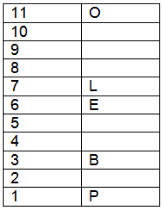Two books are kept between the books written by O and A. There are as many books between the books written by O and A as those between the books written by P and K. The book written by J is kept exactly between the books written by E and K.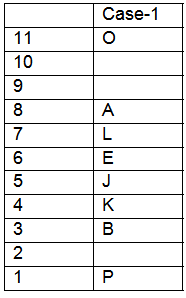The book written by P is not kept above A. The book written by I is not kept on the shelf that is numbered above 8. We get the final arrangement as given below: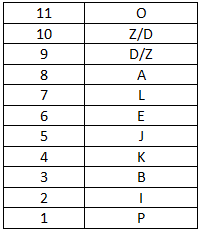So, it cannot be determined as to which author's book is kept on the tenth shelf.

SNAP Mock Test - 4 (New Pattern) - Question 19

Directions: Read the following information carefully and answer the question that follows.

Eleven books are written by different authors, named, O, D, Z, A, l, E, J, K, B, L and P. Their books are kept on different shelves. The bottom shelf is numbered 1 and the top one is numbered 11. The book written by B is kept on the third shelf. Two books are kept between the books written by O and A. There are as many books between the books written by O and A as those between the books written by P and K. The books written by O and P are on the top and the bottom shelf, respectively. The book written by L is on the seventh shelf. The book written by P is not kept above A. The book written by E is in the middle. The book written by J is kept between the books written by E and K. The book written by I is not kept on the shelf that is numbered above 8.

Which of the following combinations is/are true?

Detailed Solution for SNAP Mock Test - 4 (New Pattern) - Question 19
The book written by B is kept on the third shelf. The book written by E is in the middle. The books written by O and P are on the top and the bottom shelf, respectively. The book written by L is on the seventh shelf.Two books are kept between the books written by O and A. There are as many books between the books written by O and A as those between the books written by P and K. The book written by J is kept exactly between the books written by E and K.The book written by P is not kept above A. The book written by I is not kept on the shelf that is numbered above 8. We get the final arrangement as given below:This is true that the book written by J is kept on the fifth shelf.

SNAP Mock Test - 4 (New Pattern) - Question 20

Directions: Read the following information carefully and answer the question that follows.

Eleven books are written by different authors, named, O, D, Z, A, l, E, J, K, B, L and P. Their books are kept on different shelves. The bottom shelf is numbered 1 and the top one is numbered 11. The book written by B is kept on the third shelf. Two books are kept between the books written by O and A. There are as many books between the books written by O and A as those between the books written by P and K. The books written by O and P are on the top and the bottom shelf, respectively. The book written by L is on the seventh shelf. The book written by P is not kept above A. The book written by E is in the middle. The book written by J is kept between the books written by E and K. The book written by I is not kept on the shelf that is numbered above 8.

How many books are kept between the books written by A and K?

Detailed Solution for SNAP Mock Test - 4 (New Pattern) - Question 20
The book written by B is kept on the third shelf. The book written by E is in the middle. The books written by O and P are on the top and the bottom shelf, respectively. The book written by L is on the seventh shelf.Two books are kept between the books written by O and A. There are as many books between the books written by O and A as those between the books written by P and K. The book written by J is kept exactly between the books written by E and K.The book written by P is not kept above A. The book written by I is not kept on the shelf that is numbered above 8. We get the final arrangement as given below:Three books are kept between A and K.

SNAP Mock Test - 4 (New Pattern) - Question 21

If the current time is 6:20, then after how much time will the hands of the clock make the same angle as they do at present?

Detailed Solution for SNAP Mock Test - 4 (New Pattern) - Question 21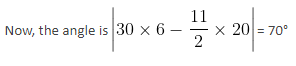If the angle between the hands is 70°, then the time can be found as follows.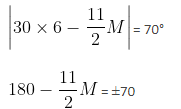M = 220/11 = 20 minutes or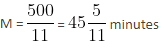So, the same angle will be made at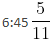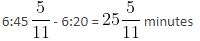SNAP Mock Test - 4 (New Pattern) - Question 22

A + B means A is the daughter of B, A × B means A is the son of B and A - B means A is the wife of B.

If P × Q - S, then which of the following is true?

Detailed Solution for SNAP Mock Test - 4 (New Pattern) - Question 22
P × Q - S means P is the son of Q who is the wife of S.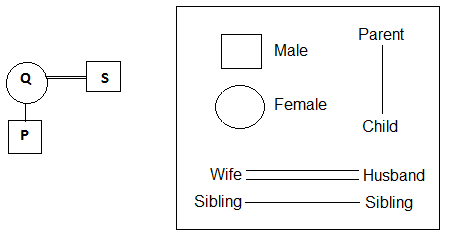From the above diagram, we can conclude that S is the father of P.

SNAP Mock Test - 4 (New Pattern) - Question 23

Directions: A machine rearranges a given input line step-by-step as per the illustration given below.

Input: 24 juice 4 manager 32 28 can tar 16 state 8 12 people 20 location ball

Step 1: ball 32 24 juice 4 manager 28 can tar 16 state 8 12 people 20 location

Step 2: ball can 32 28 24 juice 4 manager tar 16 state 8 12 people 20 location

Step 3: ball can juice 32 28 24 20 4 manager tar 16 state 8 12 people location

Step 4: ball can juice location 32 28 24 20 16 4 manager tar state 8 12 people

Step 5: ball can juice location manager 32 28 24 20 16 12 4 tar state 8 people

Step 6: ball can juice location manager people 32 28 24 20 16 12 8 4 tar state

Step 7: ball can juice location manager people state 32 28 24 20 16 12 8 4 tar

Step 8: ball can juice location manager people state tar 32 28 24 20 16 12 8 4

Step 8 is the last step of the rearrangement.

As per the rules followed in the above steps, answer the given question based on the following input:

Input: kites 30 optimistic 5 glacier watch 40 25 umbrella 20 rest 10 15 nature 35 desk

Q. Which of the following will be step 6?

Detailed Solution for SNAP Mock Test - 4 (New Pattern) - Question 23
The logic used here is explained below

For words: Words are arranged alphabetically from left to right according to English dictionary by looking at the first letter of each word. So, the first letter which comes first alphabetically among all the words is picked and written at the left end of the row. Then, the next letter is written after it. The words are put together one after another. All the words are placed at the left end of the row.

For numbers: Numbers are arranged in descending order. The largest number is picked first and after that the second largest number and so on. Numbers are arranged in such a way that they are put together with other numbers. All the numbers are arranged at the right end of the row.

The complete rearrangement is as follows.

Input: kites 30 optimistic 5 glacier watch 40 25 umbrella 20 rest 10 15 nature 35 desk

Step 1: desk 40 kites 30 optimistic 5 glacier watch 25 umbrella 20 rest 10 15 nature 35

Step 2: desk glacier 40 35 kites 30 optimistic 5 watch 25 umbrella 20 rest 10 15 nature

Step 3: desk glacier kites 40 35 30 optimistic 5 watch 25 umbrella 20 rest 10 15 nature

Step 4: desk glacier kites nature 40 35 30 25 optimistic 5 watch umbrella 20 rest 10 15

Step 5: desk glacier kites nature optimistic 40 35 30 25 20 5 watch umbrella rest 10 15

Step 6: desk glacier kites nature optimistic rest 40 35 30 25 20 15 5 watch umbrella 10

Step 7: desk glacier kites nature optimistic rest umbrella 40 35 30 25 20 15 10 5 watch

Step 8: desk glacier kites nature optimistic rest umbrella watch 40 35 30 25 20 15 10 5

From the above pattern, we see that option (1) is correct.

SNAP Mock Test - 4 (New Pattern) - Question 24

(i) A, B, C, D, E, F and G are seven members standing in a row (not necessarily in the same order) facing north.

(ii) C and B have as many members between them as G and C have between them.

(iii) D, who is 3rd from the extreme left, is 3rd to the left of E.

(iv) A and D are immediate neighbours and F and C are immediate neighbours.

Which of the following statements is true?

Detailed Solution for SNAP Mock Test - 4 (New Pattern) - Question 24
(i) A, B, C, D, E, F and G are seven members standing in a row (not necessarily in the same order) facing north.

(ii) D, who is 3rd from the extreme left, is 3rd to the left of E.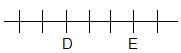(ii) C and B have as many members between them as G and C have between them.

(iv) A and D are immediate neighbours and F and C are immediate neighbours.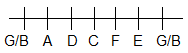SNAP Mock Test - 4 (New Pattern) - Question 25

A woman and her grandson have the same birthday. For six consecutive birthdays, her age is an integral multiple of his age. How old is the grandmother at the sixth of these birthdays?

Detailed Solution for SNAP Mock Test - 4 (New Pattern) - Question 25
Let ''d'' be the difference between the age of grandmother and grandson.

Among those six years, there will one for which the age of the grandson will be a multiple of 6.

Then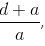(the age of the grandma) implies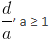Hence d is a multiple of 6. Same for 5, 4, 3, 2, 1.

Hence d is a multiple of LCM(1, 2, 3, 4, 5, 6) = 60.

Since it can't be 120, the age difference is 60.

Therefore the age of the grandson must divide 60 during those 6 consecutive years.

Meaning it must avoid all prime numbers beyond 7. But prime numbers between 7 and 89 are never more than 6 units apart.

Hence the grandson's age is 1, 2, 3, 4, 5, 6 and the age of the grandmother is 61, 62, 63, 64, 65, 66.

So at sixth birthday of grandson age of grandmother is 66 years.

SNAP Mock Test - 4 (New Pattern) - Question 26

Directions: In the problem below, a question is given followed by two arguments numbered I and II. You have to decide which of the given arguments is/are 'strong'.

Arguments:

I. No, such persons cannot serve the people and the country.

II. Yes, it is a democracy and people should be allowed to decide whom to vote for.

Q. Should persons convicted of criminal offences in the past be allowed to contest elections in India?

Detailed Solution for SNAP Mock Test - 4 (New Pattern) - Question 26
Statement I is not a valid argument; it can at best be an opinion. Hence, statement I is not strong.

Statement II is strong because it presents the logical argument; the choice of the people should be given preference.

SNAP Mock Test - 4 (New Pattern) - Question 27

Directions: In the following question, a statement is followed by two conclusions I and II. You have to take the given statement to be true even if it seems to be at variance with commonly known facts, and then decide which of the given conclusions logically follows from the statement, disregarding commonly known facts.

Statement: The Western culture is spoiling the Indian youth. With high teenage pregnancy rates and high marital dissatisfaction resulting in increasing number of divorces, the influence is deleterious on the vulnerable society of India exposed to the West through movies and other forms of social media.

Conclusions:

I. The West ought not let its culture cast its evil effect on the Indian culture in general and youth in particular.

II. Western influence through movies and social media is the only deleterious effect on Indian culture and youth.

Detailed Solution for SNAP Mock Test - 4 (New Pattern) - Question 27
I cannot be concluded as the statement talks about a problem but does not propose any solution whatsoever.

II cannot be concluded, as no mention of these being the only means of harmful influence is there in the statement.

SNAP Mock Test - 4 (New Pattern) - Question 28

Directions: Find the missing term (?) out of the options given below after studying the relationship among the other given figures.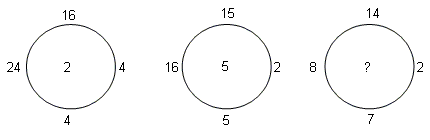Detailed Solution for SNAP Mock Test - 4 (New Pattern) - Question 28
Middle number is the difference between the quotients obtained by dividing the top number by the bottom number, and dividing the number on the left side of the circle by the number on the right side of the circle.

1. 16 ÷ 4 = 4, 24 ÷ 4 = 6, 6 - 4 = 2; 2 is the middle number

2. 16 ÷ 2 = 8, 15 ÷ 5 = 3, 8 - 3 = 5; 5 is the middle number.

3. 8 ÷ 2 = 4, 14 ÷ 7 = 2, 4 - 2 = 2; 2 is the middle number.

SNAP Mock Test - 4 (New Pattern) - Question 29

In the question below a statement is followed by two assumptions numbered I and II. An assumption is something supposed or taken in for granted. You have to consider the statement and the following assumptions and then decide which of the assumptions is implicit in the statement.

Statement: A fund promises to double the investor's money in one year.

Assumption:

I. People are looking to grow their wealth.

II. The fund is a scam.

Detailed Solution for SNAP Mock Test - 4 (New Pattern) - Question 29
Only if people want to grow their wealth would the fund be in operation. Thus, assumption I is implicit.

We cannot assume that the fund is a scam on the basis of the promise they made. Thus, assumption II is not implicit.

Hence, option A is the right answer.

SNAP Mock Test - 4 (New Pattern) - Question 30

Statements: The most expensive city in India to live is Mumbai.

Conclusions:

I. It is cheaper to live in Delhi when compared to Mumbai.

II. Indore is the cheapest city to live.

Detailed Solution for SNAP Mock Test - 4 (New Pattern) - Question 30
Since Mumbai is the costliest city, Delhi will be comparatively cheaper. Thus, conclusion I follows.

Further, we cannot conclude which city is cheaper to live. Thus, conclusion II does not follow.

Hence, option A is the right answer.

SNAP Mock Test - 4 (New Pattern) - Question 31

Statement: A company found that most of the employees in the top echelons are male. The company wants to improve the gender ratio and ensure that more female talent finds its way to the top. Which of the following arguments provide a strong reason for the company to promote gender balance?

Argument 1: Lack of women in the top echelons affects the ability of the company to make inclusive decisions.

Argument 2: The company can project itself as the best place for women to work at if the gender ratio becomes healthy.

Detailed Solution for SNAP Mock Test - 4 (New Pattern) - Question 31
Argument 1 explains how fixing the gender ratio will solve a real issue that is plaguing the company. Improving the gender ratio will help the company see things that were hidden from its sight before. Argument 1 provides a strong case for improving the gender ratio.

Argument 2 is more of a publicity stunt than a solution. Argument 2 does not explain how improving the gender ratio actually makes the company a better place. Therefore, only argument 1 is strong and hence, option A is the right answer.

SNAP Mock Test - 4 (New Pattern) - Question 32

Over the years, Traffic Police has observed a constant rise in the number of road accidents on the Chetak Bridge in winter mornings. Analysts suggest that the increase in the number of accidents is due to increase in the fog which in turn causes decreased visibility. Analysts also suggest that the increase in the fog is caused due to the increased pollution.

Courses of action:

I) Traffic Police should ask the government to ban the entry of BS III vehicles on the Chetak Bridge which causes more pollution.

II) Traffic police should ask the government to install more speed breakers on the Chetak Bridge to keep the speed of vehicles in check.

Detailed Solution for SNAP Mock Test - 4 (New Pattern) - Question 32
I is incorrect. Banning BS III vehicles on the Chetak Bridge won’t help the pollution. Banning such vehicles all together is a viable choice. But here, banning is to be done only on the Chetak Bridge. Thus, it is incorrect.

II is also incorrect. The argument doesn’t talk about deaths due to road accident. It is true that it will be unlikely that a road accident will result in a death when the vehicle is in low speed.

But here, the matter of concern is the number of accidents. Installing speed breakers won’t help in that scenario. It is a possibility that people can’t see speed breakers due to low visibility which in turn leads to accident.

Thus, D is the correct answer.

SNAP Mock Test - 4 (New Pattern) - Question 33

Given below is a statement followed by some conclusions. You have to take the given statement to be true even if it seems to be at variance with the commonly known facts and then decide which of the given conclusions logically follow(s) from the given statement.

Statement: Two planes of the A-450 series of Skyline company have crashed in the past six months raising the security concerns related to aviation.

Conclusions:

I: All the operators should ground the planes of A-450 series by Skyline company until the investigation on the mishap completes.

II: The government should ban the Skyline company from the production of any kind of planes in the future.

Detailed Solution for SNAP Mock Test - 4 (New Pattern) - Question 33
Only two planes have crashed, that too of a particular series. It would be appropriate to investigate the reasons behind the accidents. However, it would not be right to ban the company itself without deciding whether it was the company’s fault or some other reason.

Hence, option A is the correct answer.

SNAP Mock Test - 4 (New Pattern) - Question 34

If + means “subtracted by”, - means “added to”, * means “divided by”, and / means “multiplication by”, what is the value of 28 * 4 + 3 - 2 / 2 using BODMAS rule?

Detailed Solution for SNAP Mock Test - 4 (New Pattern) - Question 34
Substituting the codes we get 28 * 4 + 3 - 2/2 =

28 divided by 4 subtracted by 3 added to 2 multiplied by 2

By BEDMAS rule, we solve brackets first, then exponents, then division, then multiplication, then addition and finally subtraction.

So, we get:7 subtracted by 3 added to 2 multiplied by 2

Then 7 subtracted by 3 added to 4 = 7 +1 = 8

SNAP Mock Test - 4 (New Pattern) - Question 35

There are seven members in a family - A, B, C, D, E, F and G. Exactly two couples were present in the family. F has two daughters and a son. D is the uncle of A. E and G have a son. C is the grandfather of one of the members of the family.

How is B related to A?

Detailed Solution for SNAP Mock Test - 4 (New Pattern) - Question 35
C is a grandparent and F also is a grandparent => C and F are a couple because there are no other people in that generation.

Also, C is the grandfather and F is the grandmother.

E and G are a couple.

D is the uncle of A => B is the aunt of A

SNAP Mock Test - 4 (New Pattern) - Question 36

Identify the wrong number in the following series:

85, 94, 104, 115, 127, 140, 155, 169, 185, 202

Detailed Solution for SNAP Mock Test - 4 (New Pattern) - Question 36
85 + 9 = 94

94 + 10 = 104

104 + 11 = 115

115 + 12 = 127

127 + 13 = 140

140 + 14 = 154

154 + 15 = 169

So, 155 is the wrong number. It should be 154.

SNAP Mock Test - 4 (New Pattern) - Question 37

A series is given with one missing term. Choose the correct alternative from the given options that will complete the series.

WY, CE, IK, OQ, ?

Detailed Solution for SNAP Mock Test - 4 (New Pattern) - Question 37
W + 2 = Y

Y + 4 = C; C + 2 = E

E + 4 =I; I + 2 = K

K + 4 = O; O + 2 = Q

Q + 4 = U; U + 2 = W

Hence, option D is the right choice.

SNAP Mock Test - 4 (New Pattern) - Question 38

In each of the following questions two statements are given, and these statements are followed by two conclusions numbered (1) and (2). You have to take the given two statements to be true even if they seem to be at variance from commonly known facts. Read the conclusions and then decide which of the given conclusions logically follows from the two given statements, disregarding commonly known facts.

Statements: Some fishes are alligators. All the alligators are cats.

Conclusions:

(1) Some fishes are cats.

(2) No alligator is fish.

Detailed Solution for SNAP Mock Test - 4 (New Pattern) - Question 38
As we can check from the diagram.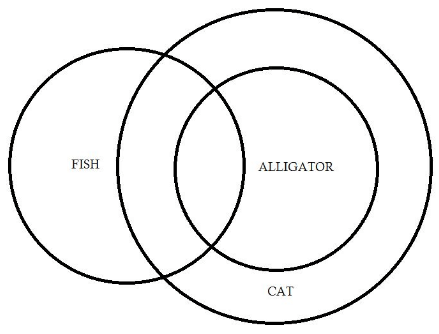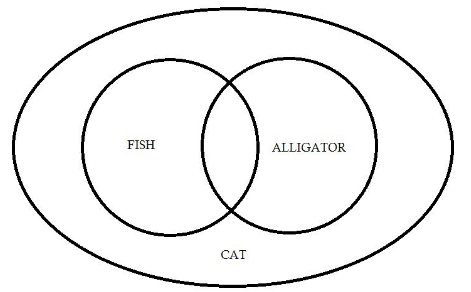Therefore, Only Statement (1) follows. Hence, Option A is correct.

SNAP Mock Test - 4 (New Pattern) - Question 39

If 15-06-1994 is wednesday, then what day is 19-07-1995 ?

Detailed Solution for SNAP Mock Test - 4 (New Pattern) - Question 39
A non leap year has 365 days (52 weeks+1 day).

1995 is not a leap year, And 15-06-1994 is wednesday, So 15-06-1995 would be thursday.

And 19-07-1995 is 34th day after 15-06-1995 (thursday), ⇒ 34 = 7(4)+6 days, So 6th day after thursday is Wednesday.

So the answer is option B.

SNAP Mock Test - 4 (New Pattern) - Question 40

At what time the hands of the clock show a mirror image of 4:10 ?

Detailed Solution for SNAP Mock Test - 4 (New Pattern) - Question 40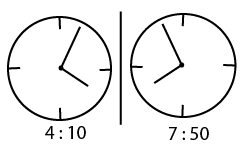It should be 7:50

So the answer is option C.

SNAP Mock Test - 4 (New Pattern) - Question 41

A train, 350 m long, travelling at a speed of 120 kmph crosses a platform in 24 sec. A man going at a certain speed crosses the platform in 3 min. What is the speed of the man?

Detailed Solution for SNAP Mock Test - 4 (New Pattern) - Question 41
Let the length of the platform be x m.

Given, length of the train = 350 m

Distance travelled = (x + 350) m

Time taken = 24 s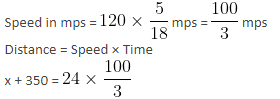x + 350 = 800

x = 450

Speed of the man = Distance/Time

Distance = 450 m

Time = 3 × 60 s = 180 s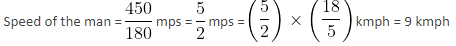SNAP Mock Test - 4 (New Pattern) - Question 42

If 200 litres of water is added to a tub that is already filled with 1200 litres of diluted milk, then the concentration of the milk in the tub will be 70%. What was the concentration of the milk in the tub before adding water to the tub?

Detailed Solution for SNAP Mock Test - 4 (New Pattern) - Question 42
Let concentration of the milk before adding water = k%

In 200 litres of water, concentration of the milk = 0%

ATQ,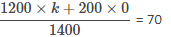⇒ 1200k = 70 × 1400

⇒ k = 81.66%

SNAP Mock Test - 4 (New Pattern) - Question 43

In how many ways can 5 persons sit on 8 chairs in a row?

Detailed Solution for SNAP Mock Test - 4 (New Pattern) - Question 43
Total number of arrangements is nPr where n = 8 and r = 5.

Required number of ways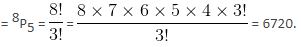SNAP Mock Test - 4 (New Pattern) - Question 44

The selling price of an article is 60% more than the profit made. If the profit on the article increases by 20%, while the selling price increases by 60%, then what is the percentage increase in cost price of the article?

Detailed Solution for SNAP Mock Test - 4 (New Pattern) - Question 44
Let initial profit on article = Rs. 100

Initial SP = 60% more than profit = Rs. 160

Initial CP = 160 - 100 = Rs. 60

Final SP = 60% more than initial SP = Rs. 256

Final Profit = 20% more than initial profit = Rs. 120

Final CP = 256 - 120 = Rs. 136

Percentage increase in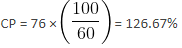SNAP Mock Test - 4 (New Pattern) - Question 45

A retailer allows a trade discount of 20% and a cash discount of 6.25% on the marked price of the products and makes a net profit of 20% on the cost. By what percentage are the products marked up by him?

Detailed Solution for SNAP Mock Test - 4 (New Pattern) - Question 45
Let MP = Rs. 100

After successive discounts,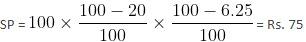CP = 75/1.2 = Rs. 62.5

Required percentage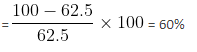Option 3 is correct.

SNAP Mock Test - 4 (New Pattern) - Question 46

Let two 8 × 12 rectangles share a common corner and overlap. The distance from the bottom right corner of one rectangle to the intersection point along the right edge of that rectangle is 7. What is the area of the shaded region?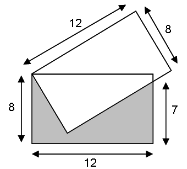Detailed Solution for SNAP Mock Test - 4 (New Pattern) - Question 46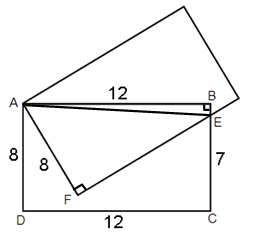Name the points as shown.

BE = BC - EC = 8 - 7 = 1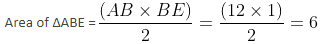Applying the Pythagoras theorem to the right triangles ABE and AFE,

AE2 = AB2 + BE2 = 122 + 12 = 145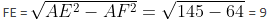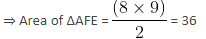⇒ Area of ABEF = 6 + 36 = 42

⇒ Area of AFECD = Area of ABCD - Area of ABEF = (8 × 12) − 42 = 54

SNAP Mock Test - 4 (New Pattern) - Question 47

In the following diagram, the line with equation y = 2x - 8 crosses the x-axis at point A and the y-axis at point B. Find the area of ΔAOB.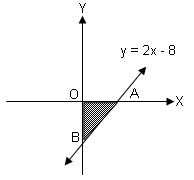Detailed Solution for SNAP Mock Test - 4 (New Pattern) - Question 47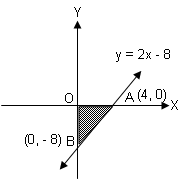Point B is the y-intercept of y = 2x - 8, so B has coordinates of (0, - 8).

Point A is the x-intercept of y = 2x - 8, so A has coordinates of (4, 0).

Therefore, area of triangle OAB = (1/2) × 4 × 8 = 16 sq. units.

SNAP Mock Test - 4 (New Pattern) - Question 48

A sum of money is lent at 8% compound interest. If the interest amount for the second year exceeds that for the first year by Rs. 32, find the sum lent

Detailed Solution for SNAP Mock Test - 4 (New Pattern) - Question 48
Let the amount lent be P.

Now, A1 = Amount at the end of the 1st year = P(1 + 0.08) = 1.08P

Interest earned in the 1st year = I1 = A1 - P = 1.08P - P = 0.08P

Now, A2 = Amount at the end of the 2nd year = P(1 + 0.08)2 = 1.1664P

I2 = Interest earned in the 2nd year = A2 - A1 = 1.1664P - 1.08P = 0.0864P

Now, 0.0864P - 0.08P = Rs. 32

Or P = Rs. 5000

Hence, option 3 is correct.

SNAP Mock Test - 4 (New Pattern) - Question 49

In a division sum, the divisor 'd' is 457, the quotient 'q' is 110 and the remainder 'r' is 242. Find the dividend.

Detailed Solution for SNAP Mock Test - 4 (New Pattern) - Question 49
Quotient (q) = 110

Divisor (d) = 457

Remainder (r) = 242

Dividend (P) = (d x q) + r

(P) = (457 x 110) + 242

P = 50270 + 242

P = 50512

Hence, the dividend is 50512.

SNAP Mock Test - 4 (New Pattern) - Question 50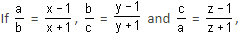then which of the following is true?

Detailed Solution for SNAP Mock Test - 4 (New Pattern) - Question 50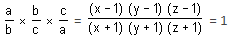⇒ (xy - x - y + 1)(z - 1) = (xy + x + y + 1)(z + 1)

⇒ xyz - xy - xz + x - yz + y + z - 1

= xyz + xy + xz + x + yz + y + z + 1

⇒ 2(xy + yz + zx + 1) = 0

∴ xy + yz + zx = -1

SNAP Mock Test - 4 (New Pattern) - Question 51

A, B and C starting running simultaneously on a circular track of length 2400 metres at speeds of 8m/s, 10 m/s and 12 m/s in the same direction. What is the minimum time after which they meet at the starting point?

Detailed Solution for SNAP Mock Test - 4 (New Pattern) - Question 51
A, B and C complete one circle in 300 s, 240 s and 200 s respectively.

So, the minimum time when they meet at the starting point is LCM (200,240,300) = 1200 secs = 20 minutes

SNAP Mock Test - 4 (New Pattern) - Question 52

Suppose A and B represent natural numbers less than 10 and the number '9874A67B' is divisible by both 9 and 11. What is the product of A and B?

Detailed Solution for SNAP Mock Test - 4 (New Pattern) - Question 52
Divisibility rule of 9: 9+8+7+4+6+7+A+B is divisible by 9 (so A+B should equal 4 or 13)

Divisibility rule of 11: |9+7+A+7| - |8+4+6+B| = 11 ⇒ A+5-B or B-A+5 is a multiple of 11..

The two divisibility rules are solvable only if A = 4 and B = 9.

The product of A and B is 36.

SNAP Mock Test - 4 (New Pattern) - Question 53

There are 15 points in a plane of which 6 are in a straight line. No three other points are on a straight line. What is the total number of triangles that can be drawn using these points?

Detailed Solution for SNAP Mock Test - 4 (New Pattern) - Question 53
The number of triangles is 15C3 - 6C3 = 435
SNAP Mock Test - 4 (New Pattern) - Question 54

How many terms are common in the series 12, 17, 22, . . . , 347 and the series 13, 20, 27, . . . , 349?

Detailed Solution for SNAP Mock Test - 4 (New Pattern) - Question 54
All terms of the form 35k - 8 are common between the two series. So, the common terms are 27, 62, 97, . . . , 342. So, the number of common terms is 10
SNAP Mock Test - 4 (New Pattern) - Question 55

A circle is inscribed in a square of side 10cm. Another circle circumscribes the square. What is the ratio of the areas enclosed between the outer circle and square to the are enclosed between the inner circle and square?

Detailed Solution for SNAP Mock Test - 4 (New Pattern) - Question 55
Diameter of inner circle = 10cm.

A1 ​ = 100 - π * 52 = 100 - 25π.

Diameter of outer circle = length of diagonal of square = 10√2.

A2 ​ = π * (5(√2)2 -100 = 50π - 100π.

Option (a) is the correct answer

SNAP Mock Test - 4 (New Pattern) - Question 56

Find the ratio of the area of quadrilateral ABDF to the area of triangle CBD.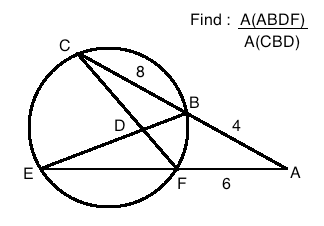Detailed Solution for SNAP Mock Test - 4 (New Pattern) - Question 56
AB*AC = AF*AE ⇒ EF = 2. Triangle CBD is similar to triangle DEF. (Angle CBD = Angle DFE: Angles subtended by the same segment and Angle CDB= Angle EDF as they are opposite angles). As lengths of corresponding segments CB and EF are in the ratio 4:1, therefore, area of triangle CBD = 16 times area of triangle EDF. Area of triangle ABD = 1/2*4*BD * sin(angle ABD)

Area of triangle CBD = 1/2 *8 * BD * sin(180 - angle ABD) = 1/2*8*BD*sin(angle ABD).

Therefore, Area of triangle ABD= area of triangle CBD / 2

Similarly, area of triangle AFD = Area of triangle DEF * 3.

Hence, A(ABDF)/A(CBD) = (A(ABD)+A(AFD))/A(CBD) = (A(CBD)/2 + 3*1/16*A(CBD))/A(CBD) = 1/2+3/16 = 11/16

SNAP Mock Test - 4 (New Pattern) - Question 57

The below graph depicts the amount spent and the amount received by a company TAL for six years. All the amounts are in lakhs.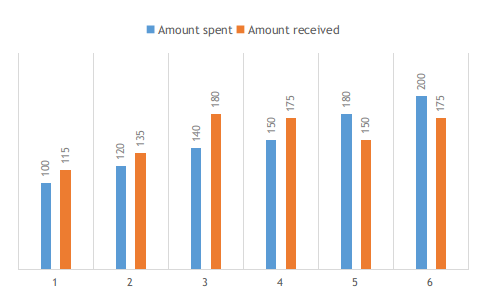The amount spent in year 5 is how much percentage less than the amount spent in year 6?

Detailed Solution for SNAP Mock Test - 4 (New Pattern) - Question 57
Amount spent in year 5 = 180 lakhs

Amount spent in year 6 = 200 lakhs

The percentage difference in the amount spent in year 5 from 6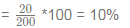SNAP Mock Test - 4 (New Pattern) - Question 58

If the sum of the roots of the equation px2 -39x + 15 = 0 is 13, then what is the product of the roots?

Detailed Solution for SNAP Mock Test - 4 (New Pattern) - Question 58
Sum of the equation = 39/ p = 13. Hence p = 3. Product of roots = 15/ 3 = 5
SNAP Mock Test - 4 (New Pattern) - Question 59

Three people are working on a project. Together, they take 10 hours to complete it. They start working together and after 5 hours, A left because he had completed his part (one-third) of the project. B also left along with A. C now takes 20 hours to finish the remaining part of the project. Find the time taken to complete the project of only B had been working on it.

Detailed Solution for SNAP Mock Test - 4 (New Pattern) - Question 59
Let the work per hour of A, B and C be a, b and c respectively.

Total work that needs to be done = 10(a+b+c). After 5 hours, work remaining = 5(a+b+c) which is done by c in 20 hours.

Therefore, 5(a+b+c) = 20c -> (1)

Since A completed one-third of the project by working for 5 hrs, we have 5a = (10/3)*(a+b+c) -> (2).

From (1) and (2), we get, a = 8b and c = 3b.

Therefore, total work involved in the project = 10*12b = 120b. Working alone, B takes 120 hours to finish it.

SNAP Mock Test - 4 (New Pattern) - Question 60

A solution of A and B contains 40 liters of A and 60 liters of B. 20% of the solution is drawn out and replaced with B. This process is carried out 4 times in total. If the final volumes of A and B are rounded to the nearest integers, what is the ratio of A:B in the final solution?

Detailed Solution for SNAP Mock Test - 4 (New Pattern) - Question 60
The final volume of A = Initial volume of A*(volume of solution that is not drawn out/total volume of the solution)n , where n is the number of times the process is repeated.

Final volume of A = 40*(80/100)^4 = 40*16*16/(25*25) = 16.384 liters

So, the ratio of A and B in the final solution = 16:84 = 4:21

## Mock Test Series for SNAP

16 docs|33 tests
Information about SNAP Mock Test - 4 (New Pattern) Page
In this test you can find the Exam questions for SNAP Mock Test - 4 (New Pattern) solved & explained in the simplest way possible. Besides giving Questions and answers for SNAP Mock Test - 4 (New Pattern), EduRev gives you an ample number of Online tests for practice

## Mock Test Series for SNAP

16 docs|33 tests

### How to Prepare for CAT

Read our guide to prepare for CAT which is created by Toppers & the best Teachers(Scan QR code)